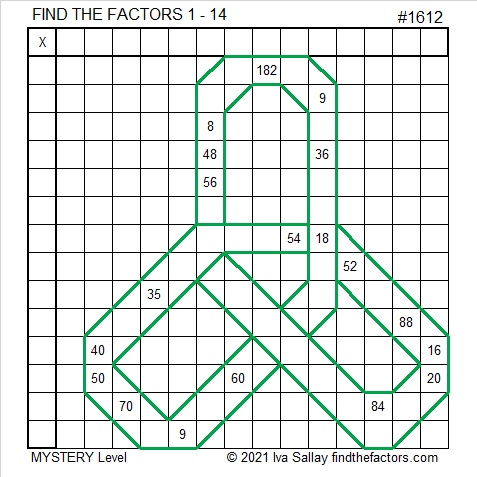# 1612 Celtic Knot

### Today’s Puzzle:

Many Celtic knots have no beginning and no end, so they are mathematically interesting. This one is like a Trinity Knot, and it doubles as a mystery-level puzzle. Solving it might be a little tricky, but it will still be lots of fun.Print the puzzles or type the solution in this excel file: 14 Factors 1604-1612.

### Factors of 1612:

• 1612 is a composite number.
• Prime factorization: 1612 = 2 × 2 × 13 × 31, which can be written 1612 = 2² × 13 × 31.
• 1612 has at least one exponent greater than 1 in its prime factorization so √1612 can be simplified. Taking the factor pair from the factor pair table below with the largest square number factor, we get √1612 = (√4)(√403) = 2√403.
• The exponents in the prime factorization are 2, 1, and 1. Adding one to each exponent and multiplying we get (2 + 1)(1 + 1)(1 + 1) = 3 × 2 × 2 = 12. Therefore 1612 has exactly 12 factors.
• The factors of 1612 are outlined with their factor pair partners in the graphic below.### More about the Number 1612:

1612 can be written as a palindromic product in two different ways:
2 × 13 × 31 × 2,
26 × 62.

1612 is the hypotenuse of a Pythagorean triple:
620- 1488-1612, which is (5-12-13) times 124.

1612 can be written as the difference of two squares in two ways:
404² – 402² = 1612, and
44² – 18² = 1612.